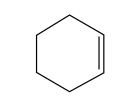# IMAT 2018 Q43 [Molecular Formula]

What is the molecular formula of the compound shown below?A. C_6H_{10}
B. C_6H_{12}
C. C_6H_8
D. C_6H_6
e. C_6H_{14}

There are a few different ways we can draw chemical formulas, and you should be familiar with recognizing them and also drawing them. This is an example of a skeletal/bond-line structure.

Skeletal/bond-line structure:

Each apex/point of the line (including empty line endings -i.e with no functional group on the end) counts as carbons. If there is a single line, it is a single carbon bond, and if there is a double or triple line, there will be a double or triple bond. We do not write out the hydrogens, but we do write out functional groups (ex. -OH).

In this example, we have a 6 carbon ring with one double bond. Normally, when we have a 6 ring with all C-C single bonds, we have 2 hydrogen per carbon, and the final formula will be C_6H_{12}. In our problem, we have a double bond, so we need to eliminate 2 hydrogens because they will be lost with the addition of a new double bond.

That changes our answer to C_6H_{10}